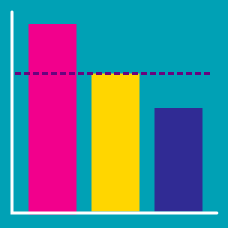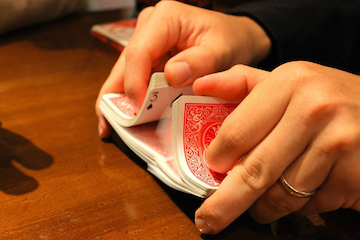Probability

# Expected Value: Level 2 Challenges

Does there exist a random variable such that the probability of it exceeding or equaling its expected value is 0?

Suppose you are sitting in an exam hall for an IQ-test containing 5 True/False questions and 5 multiple-choice questions, each having 4 different choices such that only one of the choices is right.

The probability of scoring 100% in the test by selecting options randomly is $\frac{p}{q}$ where $p$ and $q$ are integers.

$\left\lfloor \log _{ 2 }{ (p+q) } \right\rfloor \quad =\quad ?$

There are 3 envelopes, one with $1, another with$2, and the other with $4. You do not know which envelope has which amount of money. After you pick one at random, you are told which envelope of the remaining 2 has the lowest value, but not the actual value of that envelope. What is the expected value if you switch to the last envelope remaining (the one that has not yet been mentioned)?A standard deck of 52 cards is shuffled randomly, and then the cards are flipped over one-by-one. If the ace of spades is card number $S$ and the ace of hearts is card number $H$, what is the expected value of $S+H?$ A financial analyst has analyzed a new company and estimated the following information about it: The current value of the company is$1.1 million.

There is a 0.8 probability that the company will be worth $0.9 million in a year. There is a 0.15 probability that the company will be worth$1.3 million in a year.

There is a 0.05 probability that the company will be worth \$2.0 million in a year.

If the investment outlook is based on the expected future value of the company, what is the current investment outlook on the company?

×